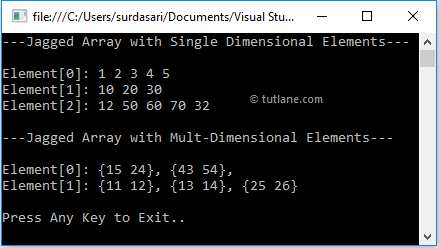# Visual Basic Jagged Arrays

In visual basic, Jagged Array is an array whose elements are arrays with different dimensions and sizes. Sometimes the a jagged array called as “array of arrays” and it can store arrays instead of a particular data type value.

## Visual Basic Jagged Array Declaration

In visual basic, the jagged array can be initialized with two brackets ()(). The first bracket will specify the size of an array and the second one will specify the dimension of an array which is going to be stored as value.

The following are the examples of creating jagged arrays in a visual basic programming language with single and multidimensional arrays.

' Jagged Array with Single Dimensional Array

Dim jarray As Integer()() = New Integer(1)() {}

' Jagged Array with Two Dimensional Array

Dim jarray1 As Integer()(,) = New Integer(2)(,) {}

If you observe the above examples, the first array (jarray) is allowed to store 2 elements of single dimensional arrays and the second array (jarray1) is allowed to store 3 elements of multidimensional arrays.

## Visual Basic Jagged Array Initialization

In visual basic, we can initialize the arrays upon declaration. The following are the different ways of declaring and initializing the jagged arrays in a visual basic programming language.

' Jagged Array with Single Dimensional Array

Dim jarray As Integer()() = New Integer(2)() {}

jarray(0) = New Integer(4) {1, 2, 3, 4, 5}

jarray(1) = New Integer(2) {10, 20, 30}

jarray(2) = New Integer() {12, 50, 60, 70, 32}

' Jagged Array with Two Dimensional Array

Dim jarray1 As Integer()(,) = New Integer(2)(,) {}

jarray1(0) = New Integer(1, 1) {{15, 24}, {43, 54}}

jarray1(1) = New Integer(,) {{11, 12}, {13, 14}, {25, 26}}

jarray1(2) = New Integer(3, 2) {}

' Initializing an Array on Declaration

Dim jarray2 As Integer()() = New Integer()() {New Integer() {1, 2, 3, 4, 5}, New Integer() {98, 56, 45}, New Integer() {32}}

If you observe the above examples, we declared and initialized the jagged arrays with single-dimensional and multidimensional arrays as an elements in different ways.

In jagged arrays the size of elements is optional. So, for some elements we didn’t mention the size to store single or multidimensional arrays with different sizes based on our requirements.

## Visual Basic Access Jagged Array Elements

In visual basic, we can access the values of jagged arrays by using row index and column index values.

Following is the example of accessing the elements from jagged arrays in visual basic programming language based on our requirements.

Dim i As Integer = jarray(0)(2) ' 3

Dim j As Integer = jarray(2)(1) ' 50

Dim k As Integer = jarray1(0)(1, 1) ' 54

Dim l As Integer = jarray1(1)(2, 1) ' 26

If you observe the above example, we are accessing the elements of single and multidimensional arrays by passing the index values.

## Visual Basic Jagged Array Example

Following is the example of using jagged arrays in visual basic programming language to represent the arrays as elements of the other array with single or multiple dimensions.

Module Module1

Sub Main()

' Jagged Array with Single Dimensional Array

Dim jarray As Integer()() = New Integer(2)() {}

jarray(0) = New Integer(4) {1, 2, 3, 4, 5}

jarray(1) = New Integer(2) {10, 20, 30}

jarray(2) = New Integer() {12, 50, 60, 70, 32}

Console.WriteLine("---Jagged Array with Single Dimensional Elements---" & vbLf)

For i As Integer = 0 To jarray.Length - 1

Console.Write("Element[{0}]: ", i)

For j As Integer = 0 To jarray(i).Length - 1

Console.Write("{0}{1}", jarray(i)(j), If(j = (jarray(i).Length - 1), "", " "))

Next

Console.WriteLine()

Next

' Jagged Array with Two Dimensional Array

Dim jarray1 As Integer()(,) = New Integer(1)(,) {}

jarray1(0) = New Integer(1, 1) {{15, 24}, {43, 54}}

jarray1(1) = New Integer(,) {{11, 12}, {13, 14}, {25, 26}}

Console.WriteLine(vbLf & "---Jagged Array with Mult-Dimensional Elements---" & vbLf)

For i As Integer = 0 To jarray1.Length - 1

Console.Write("Element[{0}]: ", i)

For j As Integer = 0 To jarray1(i).GetLength(0) - 1

Console.Write("{")

For k As Integer = 0 To jarray1(i).GetLength(1) - 1

Console.Write("{0}{1}", jarray1(i)(j, k), If(k = (jarray1(i).GetLength(1) - 1), "", " "))

Next

Console.Write("{0}{1}", "}", If(j < jarray1.GetLength(0), ", ", ""))

Next

Console.WriteLine()

Next

Console.WriteLine(vbLf & "Press Enter Key to Exit..")

End Sub

End Module

If you observe the above example, we are creating jagged array with single & multidimensional arrays and we used for loop in vb to get the elements of jagged array.

When we execute the above visual basic program, we will get the result as shown below.If you observe the above result, we stored arrays as elements in the jagged array based on our requirements.

This is how we can use the jagged arrays in our visual basic applications to store single or multidimensional arrays as an element of the jagged array.